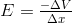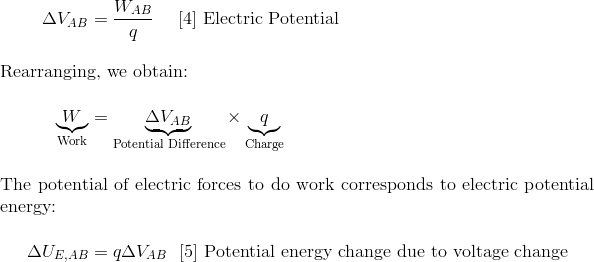Voltage

Key EquationsElectric Field Vs Electric PotentialCharge in potential energy due to travel through charging voltageElectric potential of a single charge

Vocabulary

Electrical Potential:   The amount of energy per coulomb to move a charge from one point to another in an electric field.

Electric Potential Difference / Voltage: The measured difference of the electrical potential between two points.

Guidance

Like gravity, the electric force can do work and has a potential energy associated with it. But like we use fields to keep track of electromagnetic forces, we use electric potential, or voltage to keep track of electric potential energy. So instead of looking for the potential energy of specific objects, we define it in terms of properties of the space where the objects are.

The electric potential difference, or voltage difference (often just called voltage) between two points (A and B) in the presence of an electric field is defined as the work it would take to move a positive test charge of magnitude 1 from the first point to the second against the electric force provided by the field. For any other charge, then, the relationship between potential difference and work will be:The energy that the object gains or loses when traveling through a potential difference is supplied (or absorbed) by the electric field --- there is nothing else there. Therefore, it follows that electric fields contain energy.

To summarize: just as an electric field denotes force per unit charge, so electric potential differences represent potential energy differences per unit charge. Voltage is by definition the electric potential energy per Coulomb. So it is the electrical potential energy value divided by the charge. Thus, voltage difference is the potential value for potential energy. A 12V battery can not produce energy without charge flowing (i.e. you must connect the two ends). Electric potential is measured in units of Volts– thus electric potential is often referred to as “voltage.” Electric potential is the source of the electric potential energy. You can read the electric potential lines (that is the voltage lines) just like you would a contour map while backpacking in the mountains. Positive charges move towards lower electric potential; negative charges move toward higher electric potential. Thus, positive charges go 'downhill' and negative charges go 'uphill'.

Definition Provided by CK12.org